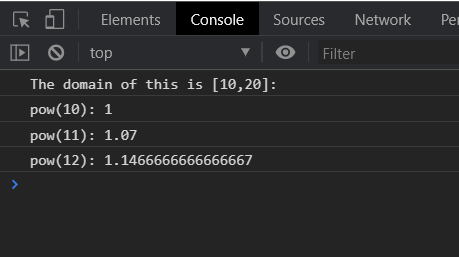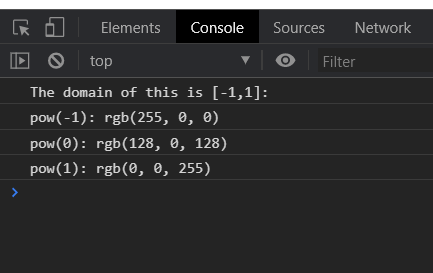# D3.js pow.domain() Function

The pow.domain() function is used to set the scale’s domain to the specified array of numbers. The array specified here must contain two or more than two elements.

Syntax:

`pow.domain([domain]);`

Parameters: This function takes a single parameter that is given above and described below.

• [domain]: An array that takes two or more values that specify the domain.

Return Value: This function does not return any value.

Example 1:

## HTML

 ` ` `<``html` `lang``=``"en"``> ` ` `  `<``head``> ` `    ``<``meta` `charset``=``"UTF-8"` `/> ` `    ``<``meta` `name``=``"viewport"` `path1tent``= ` `        ``"width=device-width,initial-scale=1.0"``/> ` `    ``<``script` `src``=``"https://d3js.org/d3.v4.min.js"``> ` `    `` ` ` ` ` `  `<``body``> ` `    ``<``script``> ` `        ``var pow = d3.scalePow() ` `         `  `            ``// Setting domain for the scale. ` `            ``.domain([10, 20]) ` `            ``.range(["1", "2", "3", "4", "5"]) ` `            ``.exponent(2); ` `        ``console.log("The domain of this is [10,20]: "); ` `        ``console.log("pow(10): " + pow(10)); ` `        ``console.log("pow(11): " + pow(11)); ` `        ``console.log("pow(12): " + pow(12)); ` `    `` ` ` ` ` `  ` `

Output:Example 2:

## HTML

 ` ` `<``html` `lang``=``"en"``> ` ` `  `<``head``> ` `    ``<``meta` `charset``=``"UTF-8"` `/> ` `    ``<``meta` `name``=``"viewport"` `path1tent``= ` `    ``"width=device-width, initial-scale=1.0"` `/> ` `     `  `    ``<``script` `src``=``"https://d3js.org/d3.v4.min.js"``> ` `    `` ` ` ` `<``style``> ` ` ` ` `  `<``body``> ` `    ``<``script``> ` `        ``var pow = d3.scalePow() ` `            ``// Setting domain for the scale. ` `            ``.domain([-1, 1]) ` `            ``.range(["red", "blue", "green", "white"]) ` `            ``.exponent(2); ` `        ``console.log("The domain of this is [-1,1]: "); ` `        ``console.log("pow(-1): " + pow(-1)); ` `        ``console.log("pow(0): " + pow(0)); ` `        ``console.log("pow(1): " + pow(1)); ` `    `` ` ` ` ` `  ` `

Output:Whether you're preparing for your first job interview or aiming to upskill in this ever-evolving tech landscape, GeeksforGeeks Courses are your key to success. We provide top-quality content at affordable prices, all geared towards accelerating your growth in a time-bound manner. Join the millions we've already empowered, and we're here to do the same for you. Don't miss out - check it out now!

Previous
Next